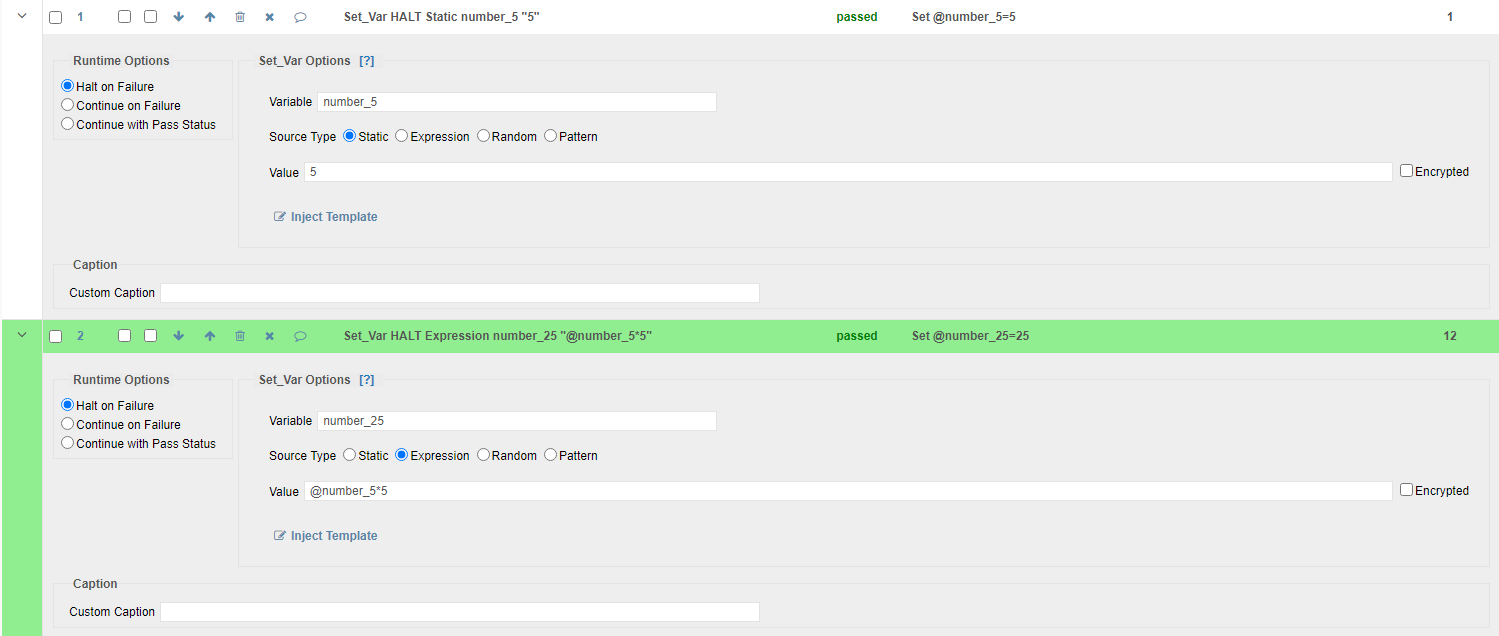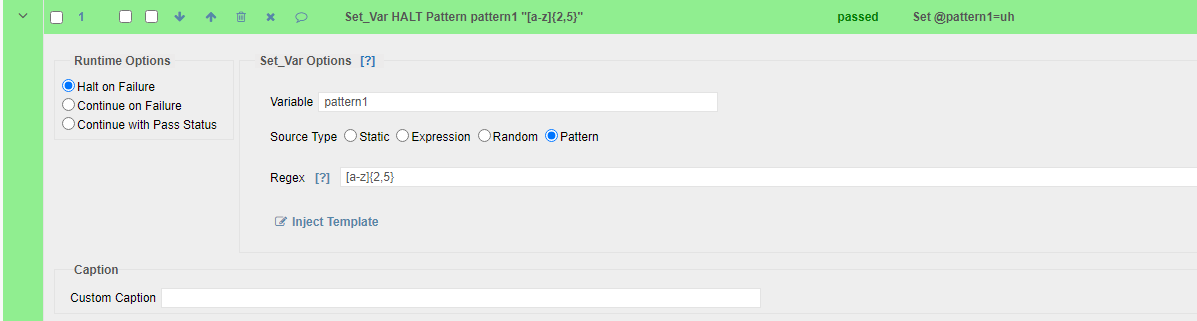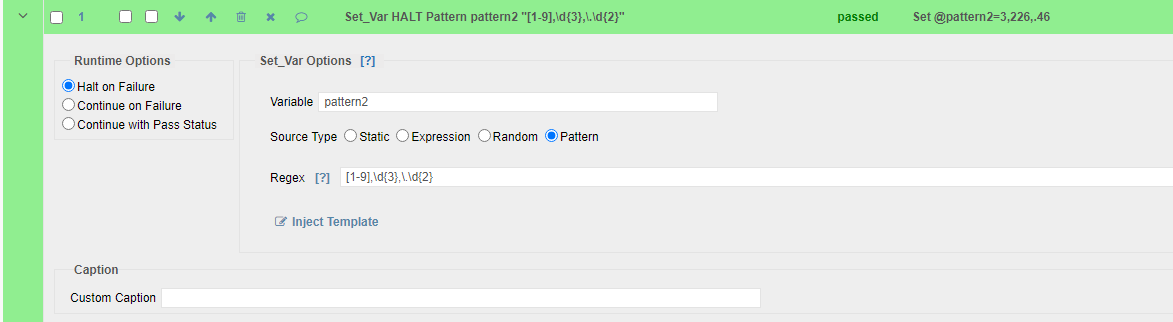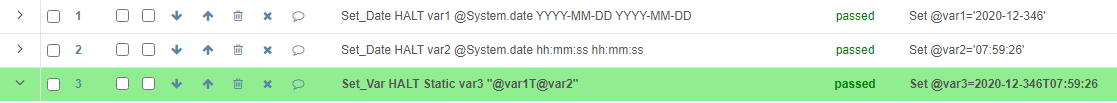# How to use expression or pattern in Set_Var command?

Can you please explain difference between expression and pattern in the set_var command?

Would you please give an example of each case?

What should we do if we want to get an variable in the format of (String)"Some fixed name identifier" +  (readable timestamp)"YYYY-MM-DDThh:mm:ss" +  (3 char random string)?

ThanksJoseph Hamdan

Hi Dhrupa,

In Set_Var/Expression, we can use other values (static or from other variables) and perform mathematical calculations.

In the example below, the first step defines a static value of 5. The second step uses this value and multiplies by 5 to produce the number 25.In Set_Var/Pattern, the regular expression is used as a pattern to generate a random value. For example '[a-z]{2,5}' or '[1-9],\d{3},\.\d{2}'

Here are some details on the example:

1- We can generate a random value from the letters [a-z] and choose the length between{2,5} as in this step:2- We can generate a random 1 digit number from 1-9 followed by random 3 digit number, a dot and a random 2 digit numberFor your last question, this is how it can be done:

1- Define a Set_Var with Static option to place your string.

2- Define a Set_Date step and use "YYYY-MM-DD" in the Source Format field. This will generate the date in which step is executed.

3- Define a Set_Date step and use "hh:mm:ss" in the Source Format field.  This will generate the time in which step is executed.

4- Define a Set_Var step which uses the variables in steps 2 and 3 and adds T in between. For example @Var_1T@Var_25- To generate 3 random character string, you have the below 2 options:

A- You can use Set_Var with Random option where you can define a length and an alphabet to choose from or add numbers if needed

B- You can use Set_Var with Pattern option and enter a Regular Expression as explained above.

6- To combine all values into one variable, you can define another Set_Var step which uses all the variables from 1, 4 and 5.

Regards,

Subject7 TeamDhrupa Patel

Great! Thanks so much!